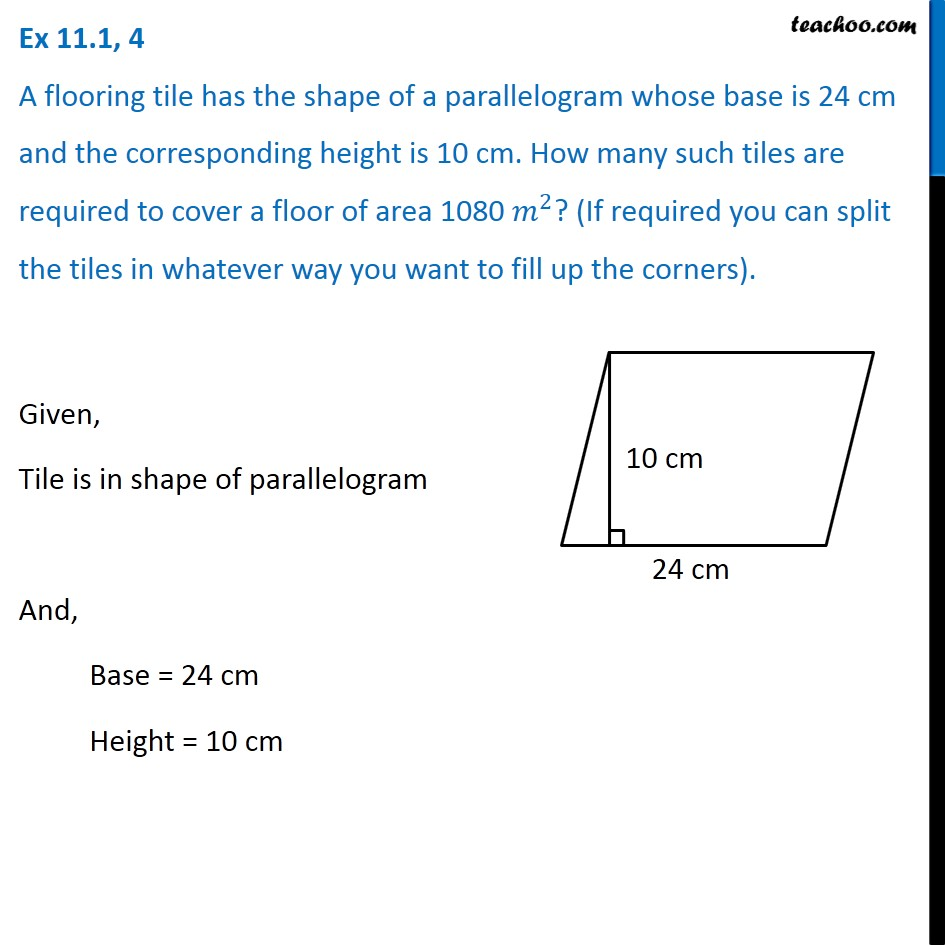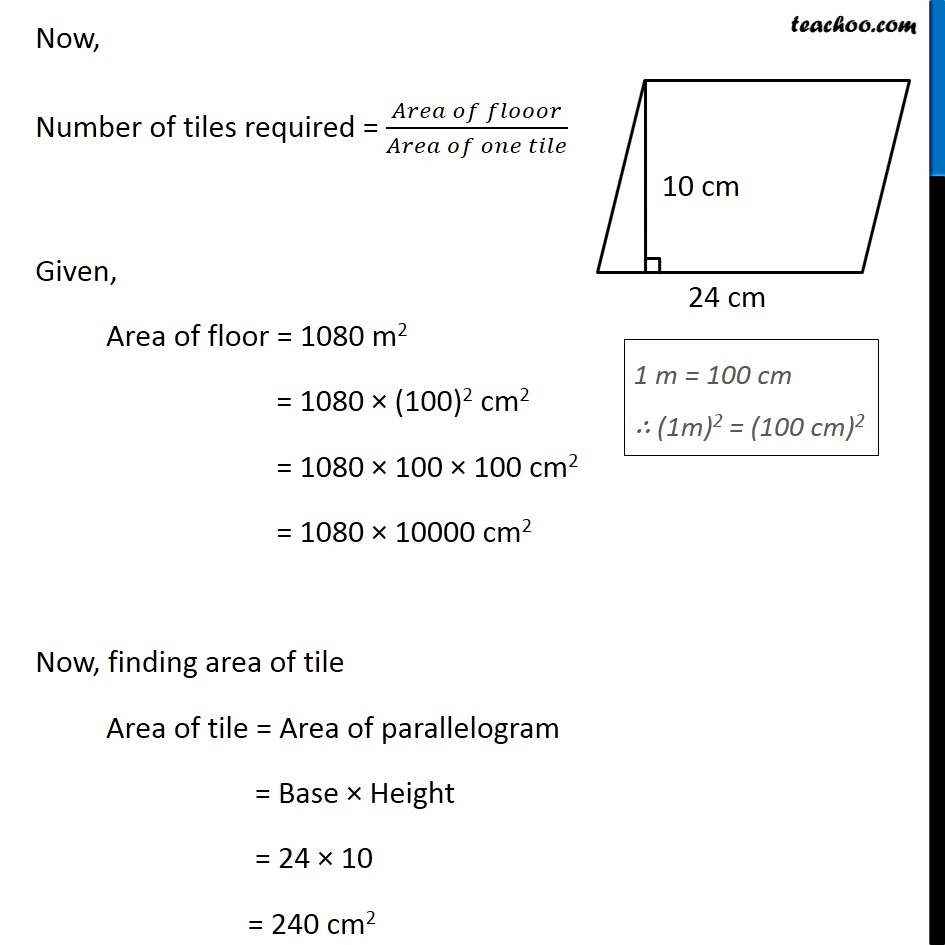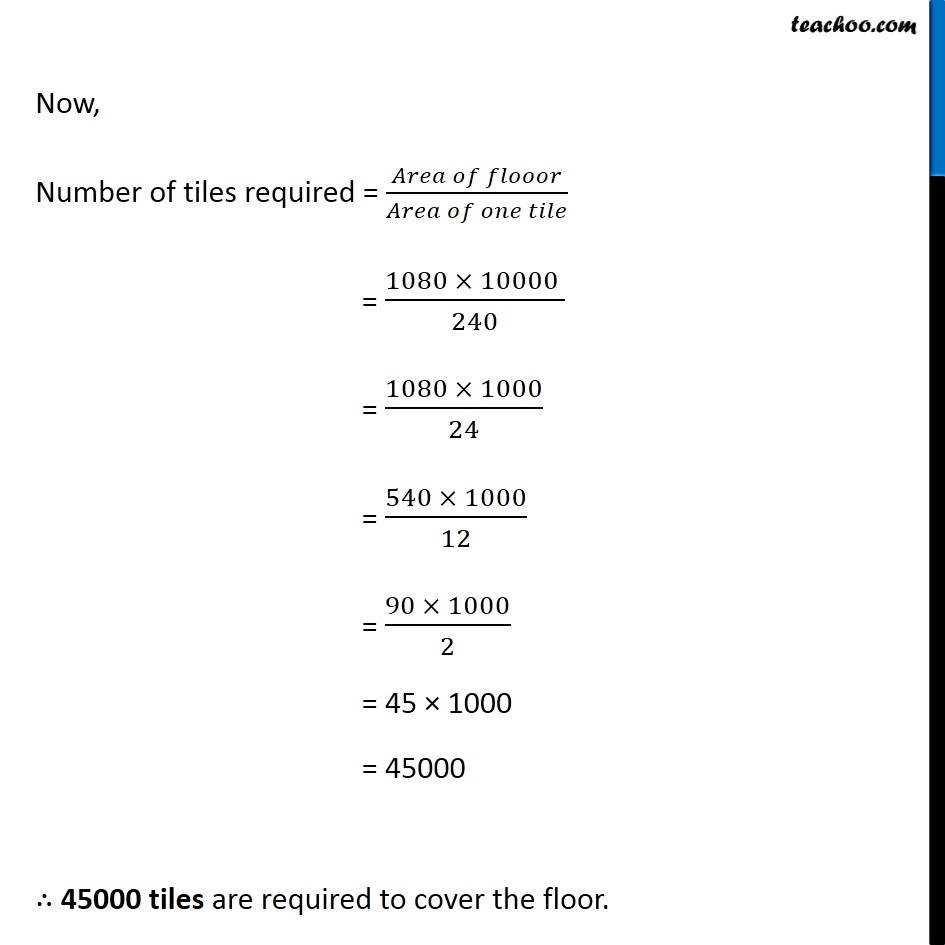Area and Perimeter

Chapter 9 Class 8 Mensuration
Concept wiseLearn in your speed, with individual attention - Teachoo Maths 1-on-1 Class

### Transcript

Question 4 A flooring tile has the shape of a parallelogram whose base is 24 cm and the corresponding height is 10 cm. How many such tiles are required to cover a floor of area 1080 𝑚^2? (If required you can split the tiles in whatever way you want to fill up the corners). Given, Tile is in shape of parallelogram And, Base = 24 cm Height = 10 cm Now, Number of tiles required = (𝐴𝑟𝑒𝑎 𝑜𝑓 𝑓𝑙𝑜𝑜𝑜𝑟)/(𝐴𝑟𝑒𝑎 𝑜𝑓 𝑜𝑛𝑒 𝑡𝑖𝑙𝑒) Given, Area of floor = 1080 m2 = 1080 × (100)2 cm2 = 1080 × 100 × 100 cm2 = 1080 × 10000 cm2 Now, finding area of tile Area of tile = Area of parallelogram = Base × Height = 24 × 10 = 240 cm2 Now, Number of tiles required = (𝐴𝑟𝑒𝑎 𝑜𝑓 𝑓𝑙𝑜𝑜𝑜𝑟)/(𝐴𝑟𝑒𝑎 𝑜𝑓 𝑜𝑛𝑒 𝑡𝑖𝑙𝑒) = (1080 × 10000 )/240 = (1080 × 1000)/24 = (540 × 1000)/12 = (90 × 1000)/2 = 45 × 1000 = 45000 ∴ 45000 tiles are required to cover the floor.